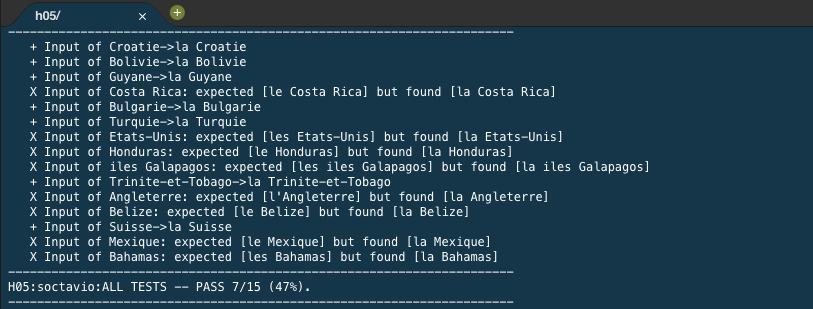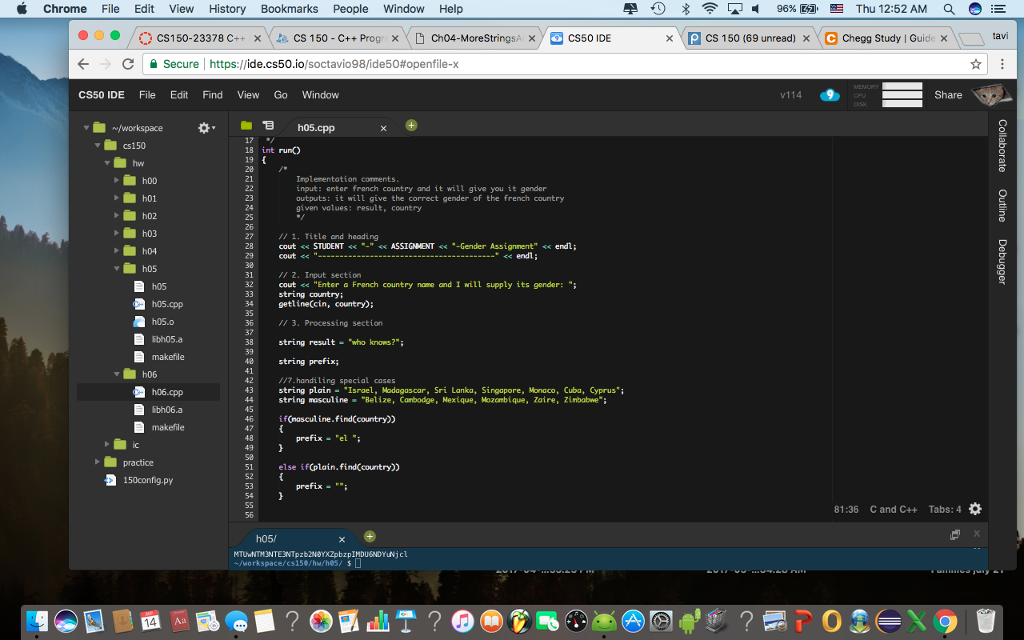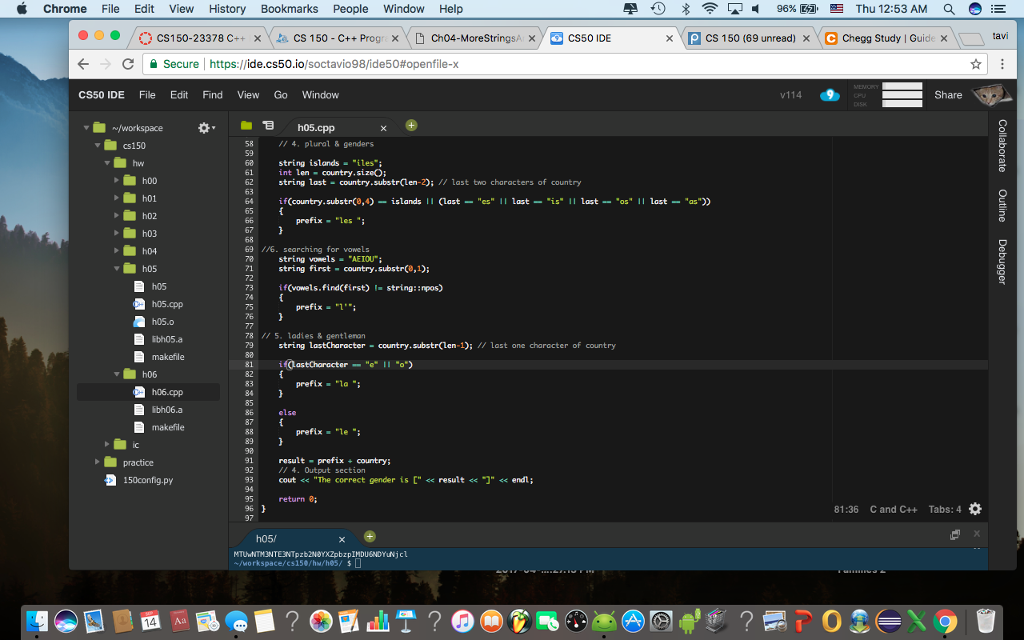# Question & Answer: h05/ Input of Croatie->la Croatie Input of Bolivie->la Bolivie Input of Guyane->la Guyane X Input of Costa Rica: expected [le Cost…..

anyone know whats wrong with my code?

int run()

Don't use plagiarized sources. Get Your Custom Essay on
Question & Answer: h05/ Input of Croatie->la Croatie Input of Bolivie->la Bolivie Input of Guyane->la Guyane X Input of Costa Rica: expected [le Cost…..
GET AN ESSAY WRITTEN FOR YOU FROM AS LOW AS \$13/PAGE

{

/*

input: enter french country and it will give you it gender

outputs: it will give the correct gender of the french country

given values: result, country

*/

cout << STUDENT << “-” << ASSIGNMENT << “-Gender Assignment” << endl;

cout << “—————————————–” << endl;

// 2. Input section

cout << “Enter a French country name and I will supply its gender: “;

string country;

getline(cin, country);

// 3. Processing section

string result = “who knows?”;

string prefix;

//7.handiling special cases

string plain = “Israel, Madagascar, Sri Lanka, Singapore, Monaco, Cuba, Cyprus”;

string masculine = “Belize, Cambodge, Mexique, Mozambique, Zaire, Zimbabwe”;

if(masculine.find(country))

{

prefix = “el “;

}

else if(plain.find(country))

{

prefix = “”;

}

// 4. plural & genders

string islands = “iles”;

int len = country.size();

string last = country.substr(len-2); // last two characters of country

if(country.substr(0,4) == islands || (last == “es” || last == “is” || last == “os” || last == “as”))

{

prefix = “les “;

}

//6. searching for vowels

string vowels = “AEIOU”;

string first = country.substr(0,1);

if(vowels.find(first) != string::npos)

{

prefix = “l'”;

}

string lastCharacter = country.substr(len-1); // last one character of country

if(lastCharacter == “e” || “o”)

{

prefix = “la “;

}

else

{

prefix = “le “;

}

result = prefix + country;

// 4. Output section

cout << “The correct gender is [” << result << “]” << endl;

return 0;

}h05/ Input of Croatie->la Croatie Input of Bolivie->la Bolivie Input of Guyane->la Guyane X Input of Costa Rica: expected [le Costa Rica] but found [la Costa Rica] Input of Bulgarie->la Bulgarie Input of Turquie->la Turquie X Input of Etats-Unis: expected [les Etats-Unis] but found [la Etats-Unis] X Input of Honduras: expected [le Honduras] but found [la Honduras] X Input of iles Galapagos: expected [les iles Galapagos] but found [la iles Galapagos] Input of Trinite-et-Tobago->la Trinite-et-Tobago X Input of Angleterre: expected [l’Angleterre] but found [la Angleterre] X Input of Belize: expected [le Belize] but found [la Belizel Input of Suisse->la Suisse X Input of Mexique: expected [le Mexique] but found [la Mexiquel X Input of Bahamas: expected [les Bahamas] but found [la Bahamas] H05: soctavio: ALL TESTS — PASS 7/15 (47%).

Given below is the fixed code.

All tests pass except Honduras. It ends with “as” and according to your condition , names ending with “as” should get prefix as “les” and that is what your code does. But the screenshot expects “la” . I am not sure if there is any exception rule for it. Please check that out.

The fixes to your code are

1. You should use if-else-if… otherwise after the if-else in beginning assigns masculine gender/plain, the last else where “le” is assigned gets executed. By make if-else if continuously , you make only one of the prefix and that will not be re-assigned in another if/else block.

2. the condition for checking masculine and plain should compare against string::npos which was not done. Also checking if lastCharacter is “o”, you had omitted the comparison operator.

I have highlighted the fixes other than making it multiple if-else if…

Since the rules are not posted in Question, I am unable to figure out how Honduras is to classified.

Hope the answer helps. If it did, please don’t forget to rate it. Thank you very much.

int run()
{
/*
input: enter french country and it will give you it gender
outputs: it will give the correct gender of the french country
given values: result, country
*/

cout << STUDENT << “-” << ASSIGNMENT << “-Gender Assignment” << endl;
cout << “—————————————–” << endl;

// 2. Input section
cout << “Enter a French country name and I will supply its gender: “;
string country;
getline(cin, country);

// 3. Processing section

string result = “who knows?”;

string prefix;

//7.handiling special cases
string plain = “Israel, Madagascar, Sri Lanka, Singapore, Monaco, Cuba, Cyprus”;
string masculine = “Belize, Cambodge, Mexique, Mozambique, Zaire, Zimbabwe”;

// 4. plural & genders

string islands = “iles”;
int len = country.size();
string last = country.substr(len-2); // last two characters of country
string vowels = “AEIOU”;
string first = country.substr(0, 1);
string lastCharacter = country.substr(len-1); // last one character of country

if(masculine.find(country) != string::npos)
{
prefix = “le “;
}

else if(plain.find(country) != string::npos)
{
prefix = “”;
}

else if(country.substr(0,4) == islands || (last == “es” || last == “is” || last == “os” || last == “as”))
{
prefix = “les “;
}
else if(vowels.find(first) != string::npos) //6. searching for vowels
{
prefix = “l'”;
}
else if(lastCharacter == “e” || lastCharacter == “o”)// 5. ladies & gentleman
{
prefix = “la “;
}
else
{
prefix = “le “;
}

result = prefix + country;
// 4. Output section
cout << “The correct gender is [” << result << “]” << endl;

return 0;
}